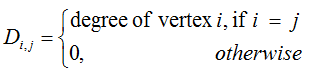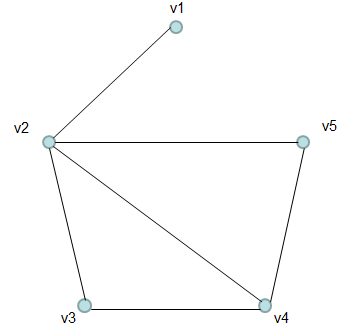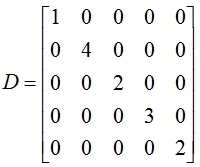Engineering Math - Quick Reference                                 Home : www.sharetechnote.com Graph Theory - Degree Matrix   Degree matrix is a diagonal matrix in which each diagonal elements indicate the degree of each vertex. (Formal defintion is as follows).For example, let's assume that we have a graph as shown below.The Degree Matrix for this graph is as follows : (Try to generate this matrix on your own based on the definition of Degree of a vertex)